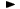ADVFN HomeHelpFinancialsKey FiguresReturn on Equity (ROE)

# Return on Equity (ROE)

Return on Equity (ROE), is a measure of a firm's profitability, expressed by the return achieved on invested equity capital. The return is therefore taken to be the attributable profit (i.e. profit after tax, minority interests and preference dividends, attributable to ordinary shareholders).

ROE is calculated as follows and is expressed as a percentage:

= (attributable profit / average ord cap,reserves) * 100